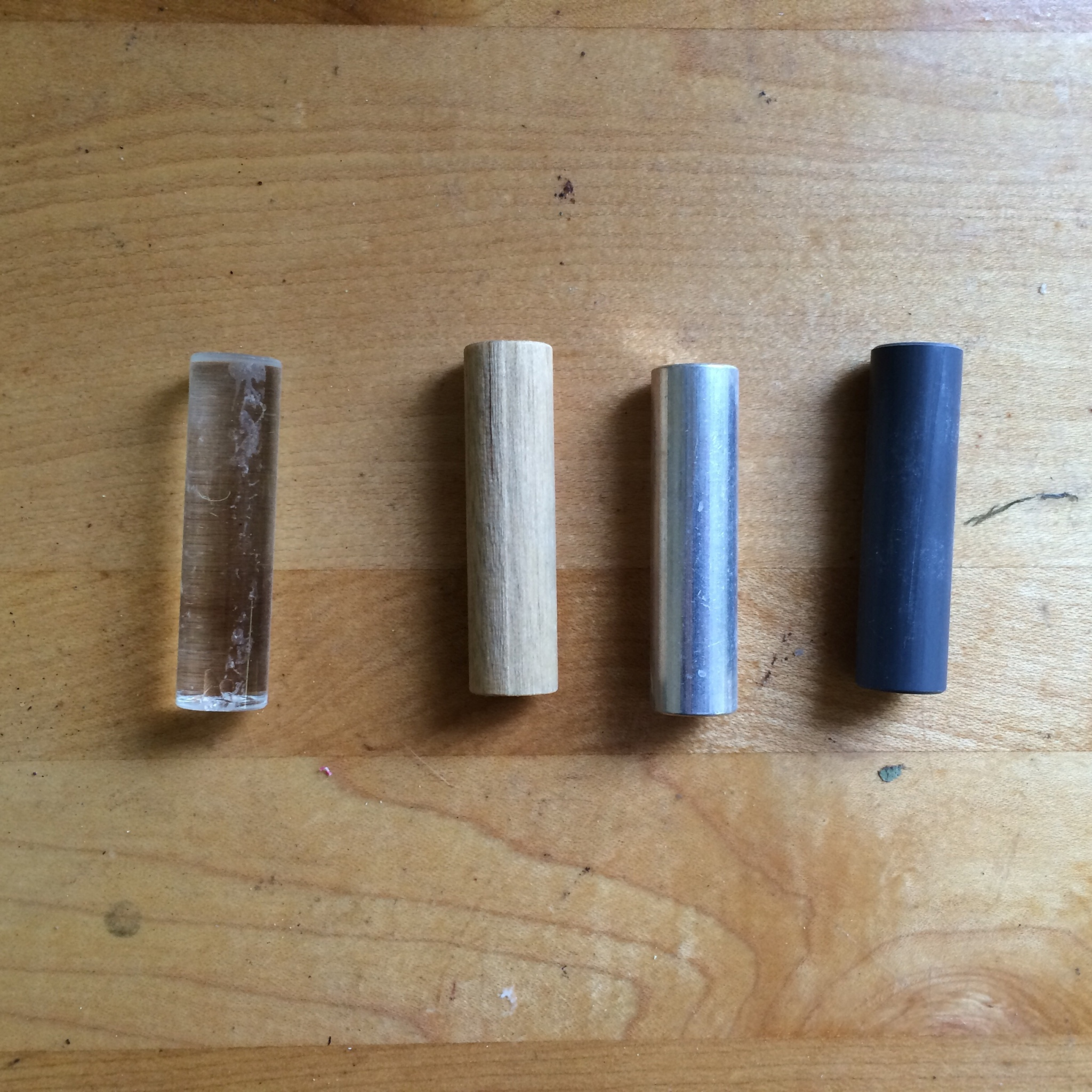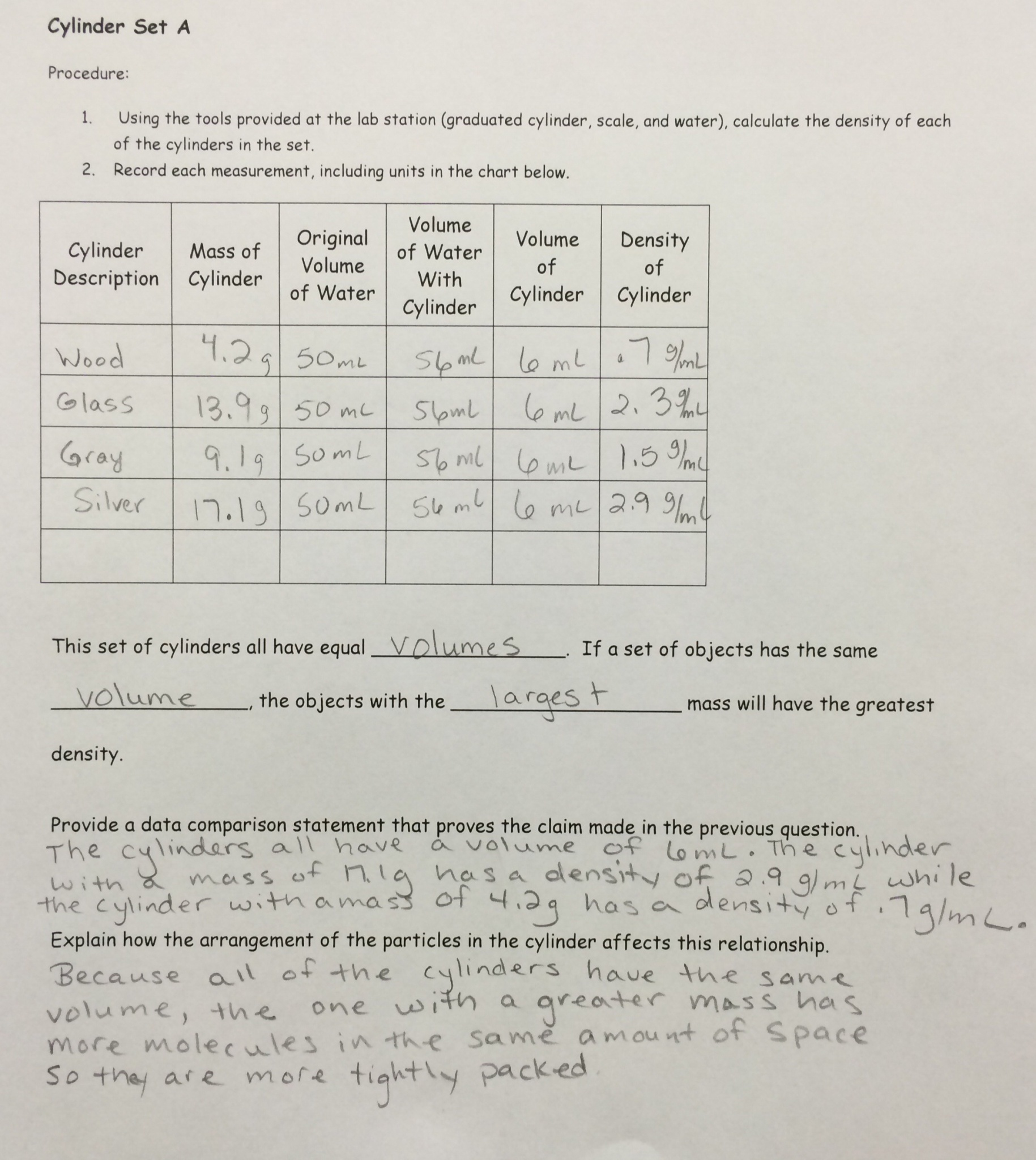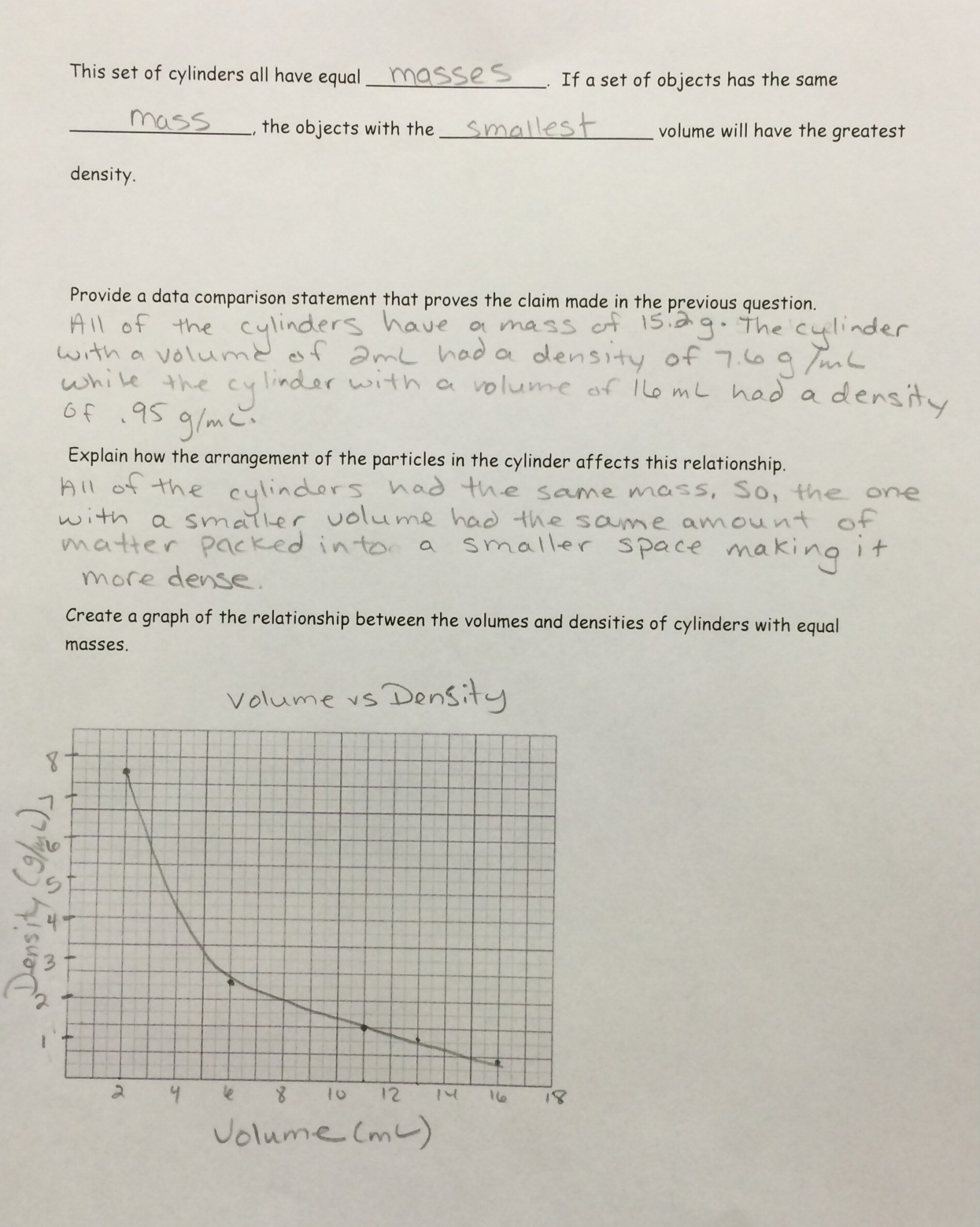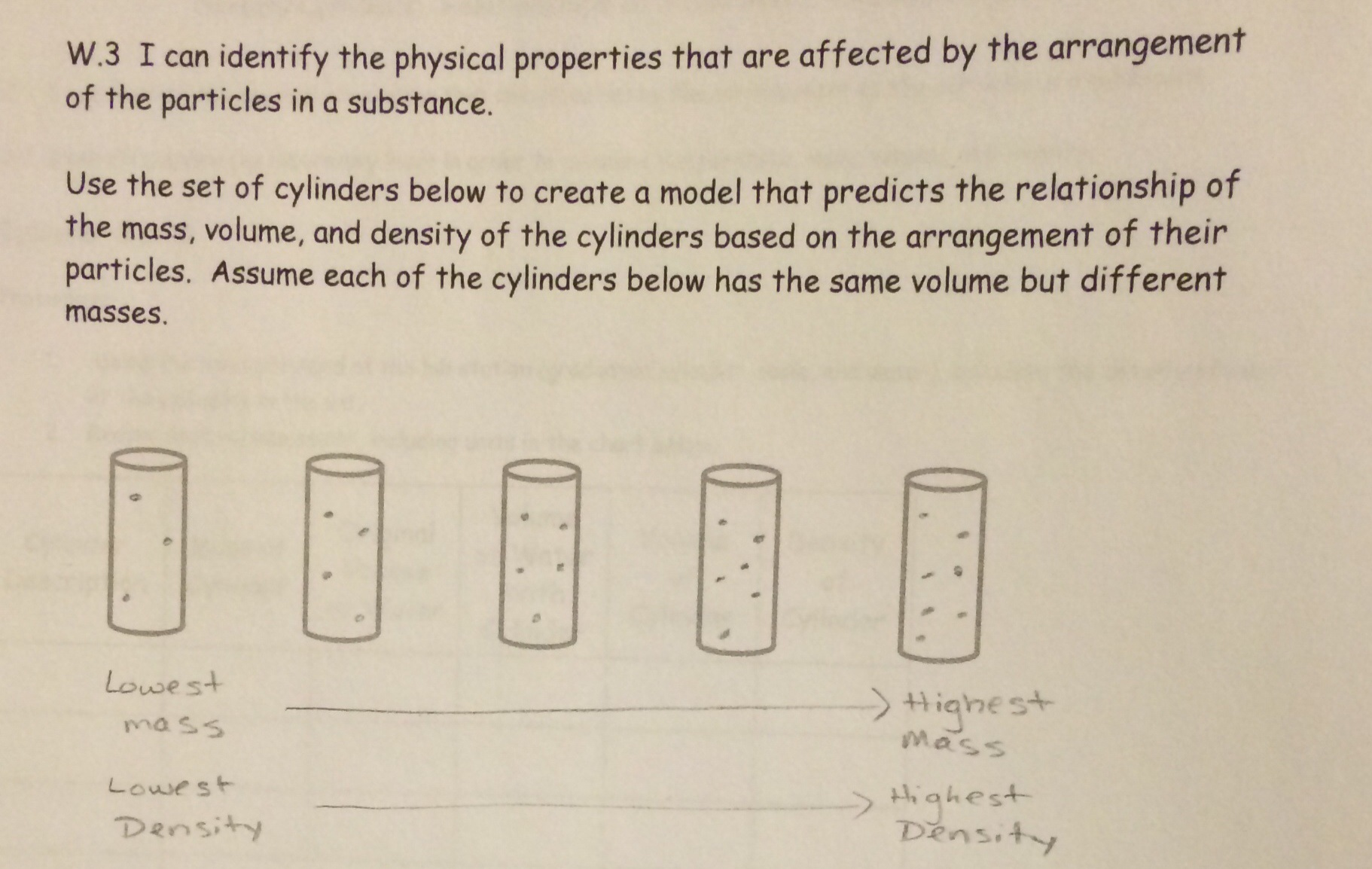# Density Cylinder Lab: An Introduction to Density

35 teachers like this lesson
Print Lesson

## Objective

Students will be able to measure the density of an object and explain the relationship between mass, volume, and density.

#### Big Idea

Students measure the density of a set of cylinders that have the same mass but different volumes to discover the relationship between mass, volume, and density!

## Introduction and Connection to the NGSS and Common Core

In this lesson, students are introduced to the idea of the measurement of physical properties.  Students are provided with two sets of cylinders.  In one set, all of the cylinders have the same mass, but different volumes.  In the other set, all cylinders have the same volume but different masses.  The focus of this lesson is not merely to calculate density, but to see patters and relationships between the variables by measuring the physical properties of the cylinders.  Students develop their conceptual awareness by realizing that in a set of objects with the same mass, the object with the smallest volume will have the largest density and within a set of objects with the same volume, the object with the largest mass with have the largest density.

In my district, students have gone through a unit on density in the previous year.  Thus, it is important to understand that this activity only involves a quick review of density and density calculations.

This lesson is designed to address the following NGSS and Common Core Standards:

MS-PS1-2  Analyze and interpret data on the properties of substances before and after the substances interact to determine if a chemical reaction has occurred.

CCSS.ELA-LITERACY.RST.6-8.3  Follow precisely a multistep procedure when carrying out experiments, taking measurements, or performing technical tasks.

CCSS.ELA-LITERACY.WHST.6-8.1.B  Support claim(s) with logical reasoning and relevant, accurate data and evidence that demonstrate an understanding of the topic or text, using credible sources.

Science and Engineering Practices:

In this lesson, students analyze and interpret data to determine the relationships between mass, volume and density from a set of collected data (SP4).

Cross Cutting Concepts:

Relating to the NGSS, students look for relationships and patterns in their data of mass and volume to determine the relationships among the variables based on the structure of the substances involve.  For example, students realize that in a set of cylinders that all have the same mass, the cylinder with the smallest volume will have the greatest density because the same amount of matter is “packed” into a smaller space.  Thus, this connects to the Cross Cutting Concept of “Patterns” which explains that macroscopic patterns are related to the nature of microscopic and atomic-level structure.

## Connecting to the Essential Question: What are you going to learn today?

5 minutes

Provide the students with the Density Unit Plan.  Explain to the students that you will be working towards answering the Essential Question, "How does the arrangement and motion of particles affect the physical properties of a substance and how can this be measured?".  Explain that in the following lessons they are going to focus on answering this.

Break down the question for the class to ensure that they understand what the question is asking.  The conversation may unfold like this:

Teacher:  What does arrangement mean?

Student:  How things are place or the order things are in.

Teacher:  Oh, that makes sense.  Sometimes I also think it is referencing how far apart objects are for each other.  What about motion?

Student:  How fast it’s moving.

Teacher:  I see it says ‘arrangement and motion of particles’.  What does that mean?

Student:  How fast and far apart particles (molecules, atoms) are.

Teacher:  Great!  So, we are focusing on how fast and far apart the molecules, particles or atoms are and how that affects physical properties.  What is a property?

Student:  A characteristic.

Teacher:  I see the word physical in front of the word property.  What do you think a physical property might be?

Student:  Like a characteristic you see physically.

Teacher:  I understand.  As I scientist, I might say that these characteristics can be measured.  Oh, and I notice that is the next part of the question, “How can they be measured?”.  So, we are trying to find out how the speed and spacing of molecules can change the characteristics of substances.  And, we have to figure out how to measure them.

Let students know that they will be focusing on skills three and four in their unit plan:

D.3  I can identify the physical properties that are affected by the arrangement of the particles in a substance.

D.4  I can effectively use laboratory tools in order to measure temperature, mass, volume, and density.

Ask students to independently take some time to underline the key vocabulary in each of these skills.  Then, in next to the skill rank themselves on their level of mastery of these skills on a scale of 1 to 4 (4 being mastery).  Let them know that it is completely fine if they are at a 1 or a 2 at this point.  This is the beginning of the unit.  The goal is that they will be increasing their learning with each lesson and will changes this score as they grow.

When using Essential Questions and “I can” statements, it is so important for the students to really understand what the question means.  Taking time to break the question down is critical to the EQ being a useful tool.  Also, at the beginning of any unit, in order to monitor student learning students need to rank their initial level of learning.

## Mini Lesson: Density Review and Introduction to Physical Properties

25 minutes

In my school district, Density is a unit that is covered in the previous grade level.  However, it is a concept that is important to review and develop further conceptual understanding.  In this unit, the students will be taking a “spin off” of density and relating it to the measurement of physical properties and how adding and removing thermal energy can affect the particles and thus the physical properties in a substance.  The reason I mention this is that in this quick mini lesson, I only quickly review how to calculate density.  If your students haven’t had experience with density, you would take more time introducing that prior to this lesson.

I provide students with this Density Reference Sheet that they have access to during the lab if they ever needed a quick refresher.  Then, as a class, I review the information on the sheet.  A couple of aspects of this discussion worth noting include:

1. When discussing the formula for density, I say, “I heart density.”  I draw a heart on the white board.  It is important that this heart comes to a point at the bottom.  Then, I say, “One of the reasons that I heart density is this.”  I then take my finger and “draw” a line through the middle of the heart, in turn erasing the middle portion of the line of the heart.  What is left is an “m” on top and a “v” on the bottom.  This is just like mass over volume!  I explain that as long as you can remember “I heart density.”, you can remember the formula! (This is demonstrated in the video below.)
2. I also discuss a “trick” with writing M, V, and D in a triangle with “M” as the top “point” and the “V” and “D” under the “M” as the “base” of the triangle.  With this tool, students can cover up the variable they are trying to calculate and it will reveal the operation that must be completed.  For example, if you cover up the “D”, it will leave the “M” above the “V”, meaning mass divided by volume.  Or if you cover up the “V”, it will leave the “M” above the “D” meaning that volume it mass divided by density.  Lastly, if you cover up the “M”, it leaves the “V” and “D” right next to each other, showing that to calculate mass one would multiple the volume by the density. (This is demonstrated in the video below.)

1. Equally as important as discussing the formula for density is describing how density is referencing how "tightly packed" the particles in a substance are.
2. When discussing the lab tools required to measure mass and volume, I hold up the tool in front of the class so that they get a clear visual of what each tool looks like.
3. When reviewing displacement, I model it for the class in a graduated cylinder.  Because it is hard to see, I draw the graduated cylinder, water, and object on the board to represent what I am seeing in the cylinder.

For my students, the “new” material lies in the idea of physical properties.  I relate back to the discussion we had when we were “breaking” down the EQ in the previous section.  The discussion might unfold like this:

Teacher:  Earlier, we were saying that a physical property that was a characteristic that you can see or measure.  And, you were completely write!  A property describes how an object looks, feels, or acts.  Physical properties can be observed or measured without changing the composition of matter (what the matter is made up of)Physical properties are used to observe and describe matter.  What do you think might be some examples of physical properties?  If I gave you a substance, what characteristics of the substance could you observe or measure?

Students brainstorm a list similar to the following:

mass, volume, density, temperature, boiling point, color, state of matter, solubility (if it can dissolve), texture, luster, etc.

**As students offer suggestions, they may not use the precise science words.  When I write their suggestions on the board, I make sure I use the science vocabulary.  For example, the student might say, “how big it is” and I might say, “Oh, you mean ‘volume’ (how much space an object takes up).

Explain that in this unit and lesson, we will focus on measuring the physical properties of temperature, mass, volume, and density and that in today’s lesson the students will be investigating the relationships between mass, volume, and density.

## Density Cylinders Lab: How do mass and volume affect density?

45 minutesProvide students with the Density Cylinders Lab Student Document.  In the document, "Cylinder Set A" is referring to the "Equal Mass Set" and "Cylinder Set B" is referring to the "Equal Volume Set".  I linked those sets to a website that you could purchase them; however, they are available on almost any science material website.

Students are divided in groups of 4 or five.  On one side of my room, I place Set A and the other side I place Set B.  At each station, I provide graduated cylinders, scales, and calculators.  Students work through the procedure in the student document on their own, switching from one side of the room to the other when they are finished with their first set.

Tips for Rotation:

1.  Prior to rotating, students must show me their data table.  If there are one or two cylinders whose densities are off, I send them back to measure again.

2.  I let students know that after they collect their data for one set and rotate to the next set and there are no available stations, the students can begin answering questions for the set they already completed while they wait.

3.  Also, I encourage teamwork here.  If there are five students in a group, each student can calculate the density of one cylinder.

4.  As students work, check in with each group and take note of any student that may be struggling with the measurements and provide those students with some one on one instruction.

## A Look at Student Work

Here is a quick visual of the work students complete.  The video at the bottom goes through the important aspects of the student work in detail.## Closure: Formative Assessment

5 minutes

Provide the students with this Formative Assessment - Cylinders.  Have the students complete this independently.  I provide feedback on these formative assessments and then sort the student work into groups of similar learners.  Then, in a future lesson, I conference with these groups of learners and meet with them about their similar needs.In this example, the student indicated the spectrum of mass and density, thus creating a visual model that shows the relationship between mass and density.  Students will do this in a variety of ways.  Some, will "make up" a mass for each cylinder and actually write numbers below the cylinders.  Others will use words.  The great thing about a model is that students can demonstrate understanding in a variety of ways.  What you want to look for is that they have indicated both mass and density so that the relationship is clear.  Students can tend to show one but not the other.  In many cases, these students understand the relationship but just forget to include both variables.  I conference with these students about the necessity for comparison when trying to show a relationship.  Both variables in the relationship need to be shown.

Also, notice that the student added dots in the cylinders to represent the particles.  This is very important.  Many students forget to include this in their model, although it asks for it in the question.  I conference with these students about how to determine the purpose of the question.  Another key point is that you as a teacher count the dots in the cylinders.  Simply adding dots in the cylinder isn't enough to show understanding.  For example, in this example, the student added 3 dots in the lowest mass, 4 in the next, 5 in the following, all the way up to 7 in the highest mass cylinder.  This shows that the student understands that a cylinder with a higher mass will have more particles in the same space.  If students just put a random number of dots, it shows that they broke down the question well to identify the purpose of the question; however, they were unable to model how the arrangement of the particles is related to density.

Typically, I conference with the following groups of learners based on this formative assessment:

1.  Students that forget to add particles.

2.  Students that only show one of the variables (mass or volume).

3.  Students that add particles, but add random numbers as opposed to an increasing number for the highest mass.

4.  Students that incorrectly identify the relationship (they state that more mass has a lower density).